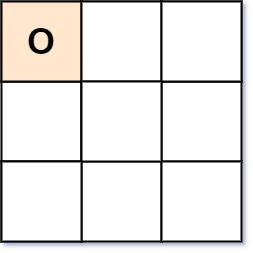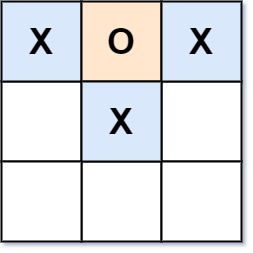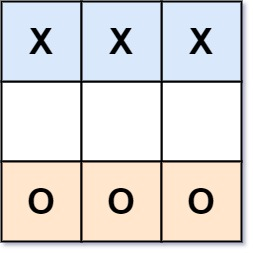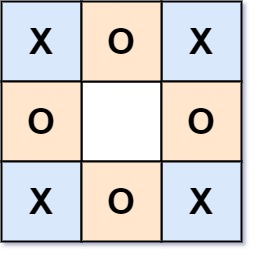# GeetCode Hub

Given a Tic-Tac-Toe board as a string array `board`, return `true` if and only if it is possible to reach this board position during the course of a valid tic-tac-toe game.

The board is a `3 x 3` array that consists of characters `' '`, `'X'`, and `'O'`. The `' '` character represents an empty square.

Here are the rules of Tic-Tac-Toe:

• Players take turns placing characters into empty squares `' '`.
• The first player always places `'X'` characters, while the second player always places `'O'` characters.
• `'X'` and `'O'` characters are always placed into empty squares, never filled ones.
• The game ends when there are three of the same (non-empty) character filling any row, column, or diagonal.
• The game also ends if all squares are non-empty.
• No more moves can be played if the game is over.

Example 1:```Input: board = ["O  ","   ","   "]
Output: false
Explanation: The first player always plays "X".
```

Example 2:```Input: board = ["XOX"," X ","   "]
Output: false
Explanation: Players take turns making moves.
```

Example 3:```Input: board = ["XXX","   ","OOO"]
Output: false
```

Example 4:```Input: board = ["XOX","O O","XOX"]
Output: true
```

Constraints:

• `board.length == 3`
• `board[i].length == 3`
• `board[i][j]` is either `'X'`, `'O'`, or `' '`.

class Solution { public boolean validTicTacToe(String[] board) { } }